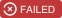# polylearn¶

A library for factorization machines and polynomial networks for classification and regression in Python.

Github repository.Factorization machines and polynomial networks are machine learning models that can capture feature interaction (co-occurrence) through polynomial terms. Because feature interactions can be very sparse, it’s common to use low rank, factorized representations; this way, we can learn weights even for feature co-occurrences that haven’t been observed at training time.

Factorization machines are popular for recommender systems, as they are a generalization of matrix completion models.

This package provides:

• coordinate descent algorithm for fitting factorization machines of degree 2 or 3,
• coordinate descent algorithm for fitting polynomial networks of arbitrary degree,
• scikit-learn-compatible API,
• Cython implementations for computationally intensive parts.

## Installation¶

Binary packages are not yet available.

The development version of polylearn can be installed from its git repository. In this case it is assumed that you have a working C++ compiler.

1. Obtain the sources by:

```git clone https://github.com/scikit-learn-contrib/polylearn.git
```

1. Install the dependencies:

```# via pip

pip install numpy scipy scikit-learn nose
pip install sklearn-contrib-lightning

# via conda

conda install numpy scipy scikit-learn nose
conda install -c conda-forge sklearn-contrib-lightning
```
2. Build and install polylearn:

```cd polylearn
python setup.py build
sudo python setup.py install
```